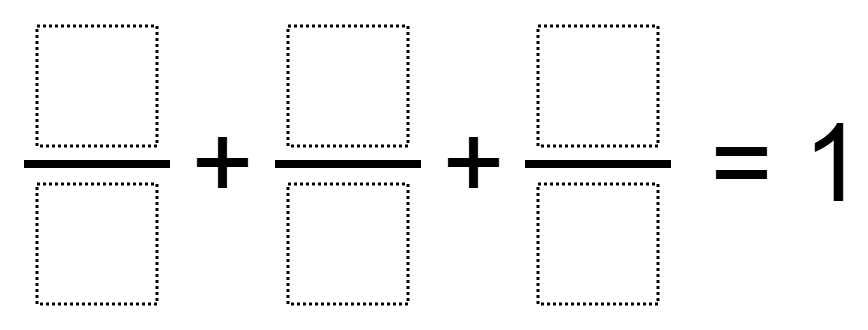# Adding 3 Fractions to Get 1

Directions: Using the digits 1 to 9, at most one time each, place a digit in each box to make a true statement.### Hint

What digits would be bad choices for the denominators?
What digits would be good choices for the denominators?

2/8 + 1/4 + 3/6 = 1
2/4 + 3/9 + 1/6 = 1 (found by Koharu in Ms. Wilson’s class)

Source: Owen Kaplinsky

## Equivalent Expressions with Fractions

Directions: Using the digits 0 to 9 at most one time each and choosing either …

1.•can only use each digit one time

•1/3 + 1/2 + 1/6

•1/3+1/3+1/3=1

2.I there only one answer? 1/4+2/8+3/6

3.Is there only one answer? 1/4+2/8+3/6

4.My students also found 4/8 + 3/9 + 1/6

5.•4/8 + 3/9 + 1/6

6.1/3 + 1/3 + 1/3 =1

7.1/3 + 1/3 + 1/3

8.2/8 + 1/4 + 3/6 = 1

9.Mikayla Malmquist

2/8 + 1/4 + 3/6 = 1

10.11.2/8+1/4+3/6

12.2/8+5/8+1/8

13.1/4 + 2/8 + 3/6 = 1

14.15.1/3+1/3+1/3

16.17.Hey Owen, this is nearly identical to the task I put up about 3 years ago, do you think we should take mine down or maybe collaborate? Your title is definitely better and I went all the way to 10 just because it provided a few more answers and touched on decimals. Also, I was inspired by the book The Wishing Club, which goes perfectly with these tasks.

•This answer does not follow the rules with 1 digit in each box, but definitely answers the question. My student came up with 9/12 + 5/34 + 7/68

•Oh! I didn’t realize that this is identical to yours! I think I should take mine down instead, because yours is the first one up. What do you think?

•I would definitely leave yours up or we’d lose this great discourse thread!

18.This answer does not follow the rules with 1 digit in each box, but definitely answers the question. My student came up with 9/12 + 5/34 + 7/68

•Gina, you should create that one and post it. It may already be on here already but by putting 1 box for numerator and 2 for each denominator you’ve created a pretty challenging task! I wonder if there is more than one answer?

•Amazing!! How could you find the answer?? Just keep trial and errors??

19.i got 1/4 + 2/8 + 3/6

20.21.1/4+2/8+3/6

22.1/4+2/8+3/6

23.1/3, 1/3, 1/3 🙂

24.3/9, 2/4, 1/6

25.2/5 + 2/5 + 1/5 = 1

•Wait no I’ll use
2/8 + 1/4 + 3/6
Because I can only use them once

26.Your friend who says I like your cut G way too much

2/8+1/4+3/6?

27.1/2 + 2/8 + 1/4 = 1

28.HELP!!!!!!

29.Nicolette Schlachter

4/8 + 3/9 + 1/6

30.My fifth-grade student, Yuel, came up with 3 possibilities:

1/4 + 3/6 + 2/8 = 8/8

1/6 + 2/4 + 3/9 = 6/6

4/8 + 3/9 + 1/6 = 6/6

31.32.I need 3 numbers that have 1 as a numerator and all different denominators! HELP!

33.1/6 + 4/8 + 3/9 =1
G.D.